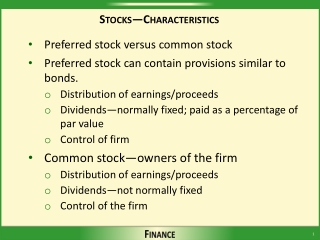DownloadDownload PresentationStocks—Characteristics

# Stocks—Characteristics

Télécharger la présentation## Stocks—Characteristics

- - - - - - - - - - - - - - - - - - - - - - - - - - - E N D - - - - - - - - - - - - - - - - - - - - - - - - - - -
##### Presentation Transcript

1. Preferred stock versus common stock Preferred stock can contain provisions similar to bonds. Distribution of earnings/proceeds Dividends—normally fixed; paid as a percentage of par value Control of firm Common stock—owners of the firm Distribution of earnings/proceeds Dividends—not normally fixed Control of the firm Stocks—Characteristics

2. Stock value = Present value of the dividends that the company is expected to pay during its life. If the stock never pays a dividend—whether a regular dividend or a liquidating dividend—then its value is \$0. Stocks—Valuation

3. 1 2 0 3 ∞ … . . . Stock ownership entitles the investor to the future cash flows, called dividends, that are paid by the firm Stocks—Valuation

4. Stocks—Valuation rs = required return on stock

5. If growth is constant such that g = g1 = g2 = … = g∞ … Valuation—Constant Growth, g Value of a constant-growth stock Required: g = constant rs > g

6. Valuation—Constant Growth, g Example: The most recent dividend paid (D0) by a firm was \$2; the firm is expected to grow at a constant rate (g) equal to 4 percent; and the required rate of return (rs) on similar risk investments is 12 percent.

7. Year Dividend, = \$2.00(1.04)t PV of @ 12% Valuation—Constant Growth, g D0 = \$2; g = 4%; rs = 12%

8. D D D D D ˆ … = + + + = = P 0 ¥ 1 2 r r + + + (1 r ) (1 r ) (1 r ) s s s s s When g = 0, the same dividend is paid each year, which is the characteristic of preferred stock Valuation—Constant Growth, g g = 0

9. Example: The preferred stock of a company pays a constant dollar dividend equal to \$4 per share. The required rate of return on similar risk investments is 8 percent. Valuation—Constant Growth, g

10. Valuation—Nonconstant Growth gnorm = constant, or normal, growth Assume constant growth, gnorm, begins at some point in the future.

11. Example: D0=\$1.25 g1= 25% g2= 20% g3= 10% g4= -4% g5= 5% = g6 = … = g∞ rs=14% nonconstant growth Valuation—Nonconstant Growth Constant growth, gnorm

12. Step 1: • Start computing dividends. • Continue computing dividends until you compute the last nonconstant growth dividend. • Find the PVs of the nonconstant growth dividends. • Sum the results. Valuation—Nonconstant Growth Steps to Follow

13. Step 2: • Compute the first constant growth dividend. • Use this dividend to compute the value of the stock at the end of the nonconstant growth period, which is the PV of all the future dividends that will grow at a constant rate, gnorm. • Compute the PV of the future stock value (price). Valuation—Nonconstant Growth Steps to Follow

14. Step 3: • Sum the PV of the nonconstant growth dividends computed in Step 1 and the PV of the future stock price computed in Step 2. Valuation—Nonconstant Growth Steps to Follow

15. D0 = \$1.25; rs = 14% Year Growth rate, g Dividend, PV of @ 14% 2 20 1.8750 = 1.5625(1.20) 1.4428 3 10 2.0625 = 1.8750(1.10) 1.3921 4 -4 1.9800 = 2.0625(0.96) 1.1723 S = 5.3778 Valuation—Nonconstant Growth Step 1: Compute PV of nonconstant-growth dividends 1 25% \$1.5625 = \$1.2500(1.25) \$1.3706

16. Because the dividends grow at a constant rate afterYear 4, we can apply the constant growth model to compute the PV of the future constant-growth dividends at the end of Year 4: Valuation—Nonconstant Growth Step 2: Compute PV of constant-growth dividends

17. Valuation—Nonconstant Growth PV of future price, = \$13.6771 PV of nonconstant dividends = \$5.3778 = Current value of the stock = \$5.3778 + \$13.6771 = \$19.05

18. 0 1 2 3 4 19.0549 Valuation—Nonconstant Growth Cash Flow Timeline 14% 1.5625 1.8750 2.0625 1.9800 5.3778 13.6771 23.10 = PV of constant growth dividends in Year 5 through Year 

19. Valuation—Nonconstant Growth The key to computing the value of a stock that exhibits nonconstant growth is to assume constant growth occurs at some point in the future—it might start in five years, 50 years, or 100 years.

20. ˆ D ˆ 1 = + r g s P 0 Valuation—Stock Returns Expected dividend yield Expected growth rate (capital gains yield) Expected rate of return = +

21. Valuation—Factors that Affect Prices • Market conditions • Investors’ risk preferences, which determine rs • Higher rs, lower price • Company factors • Growth rate • Higher g, higher price • Dividend payments • Higher D0, higher price

22. Valuation—Alternative Techniques • P/E ratios • P/E ratio = Price ÷ EPS = price multiple • “Normal” P/E • Economic value added (EVA) • Earnings must be sufficient to pay those who provide funds to the firm; otherwise the value of the firm should decrease.

23. Chapter 7 Questions What is equity? What are some characteristics of equity? Explain the following statement: “Whereas a bond contains a promise to pay interest, common stock provides an expectation but no promise of dividends.” What is the significance of the par value of a preferred stock? Of a common stock? How are stock prices determined? How are stock returns determined? What two components are included in a stock’s return? What factors affect stock prices? Explain. What alternative techniques do investors use to value stocks? Why?